﻿ 一类链环的Jones多项式 The Jones Polynomials of Class of Knots

Vol. 08  No. 05 ( 2019 ), Article ID: 30571 , 12 pages
10.12677/AAM.2019.85117

The Jones Polynomials of Class of Knots

Wei Zheng, Dongyu Geng, Luying Zhao*

Liaoning Normal University, Dalian LiaoningReceived: May 9th, 2019; accepted: May 24th, 2019; published: May 31st, 2019ABSTRACT

Knot theory is a branch of mathematical topology and the classification of knots is a very important problem in the field of knots. People solve the problem of knot by looking for knot invariants. Knot invariants are trichrome, crossover number, knot number, bridge number, knot polynomial, knot group, etc. In this paper, we study a class of special Brunnian link. The formula for calculating the Jones polynomial of this kind of chain is given. In order to realize the calculation of $B\left({c}_{1},{c}_{2},\cdots ,{c}_{n}\right)$ Jones polynomials, on the one hand, by calculating $B\left({c}_{1}\right)$ and $B\left({c}_{1},{c}_{2}\right)$ bracket polynomials, the calculating formulas of $B\left({c}_{1},{c}_{2},\cdots ,{c}_{n}\right)$ bracket polynomials are obtained. On the other hand, by dividing the given orientation into regions $B\left({c}_{1},{c}_{2},\cdots ,{c}_{n}\right)$, the enumeration method is used to find the calculation rule of the number of twists, and then the calculation formula of the number of twists $B\left({c}_{1},{c}_{2},\cdots ,{c}_{n}\right)$ is given. Combining the above two aspects, the calculation formula of the Jones polynomial $B\left({c}_{1},{c}_{2},\cdots ,{c}_{n}\right)$ is obtained. The innovation of this paper is to generalize the original definition of connected sum, to connect with a specific segment, $B\left(0\right)$ to construct $B\left(0,0\right)$, then $B\left(0,0\right)$ to construct $B\left(0,0,0\right)$, and then to find the $B\left(0,0,\cdots ,0\right)$ computational law, which greatly simplifies the computational process.

Keywords:Pretzel Knot, Jones Polynomial, Twist, Brunnian Link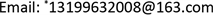Jones多项式是纽结理论中重要的不变量之一。若两个链环投影图的Jones多项式的不同，则这两个投影图对应着不同的链环，这是研究纽结和链环分类问题的重要方法。Brunnian link是一类既特殊又简单的链环，本文研究一类特殊的Brunnian链 $B\left({c}_{1},{c}_{2},\cdots ,{c}_{n}\right)$ 环，本论文给出了 $B\left({c}_{1},{c}_{2},\cdots ,{c}_{n}\right)$ 的Jones多项式的计算公式。1. 引言

2. 预备知识

2.1. 纽结及投影图

①只有有限多个交叉点；

②每个交叉点都是二重点；

③在每个二重点处，上下两线的投影都是互相穿越交叉的则称为纽结投影图。注意，平面的选择不同随之投影图不唯一。

2.2. 链环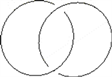2.3. Reidemeister Move (R变换)

Reidemeister变换是改变纽结的正则投影图的三种方式，每一种都会改变交叉点之间的关系 

RI变换：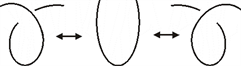RII变换：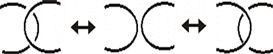RIII变换：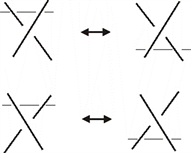2.4. 纽结连通和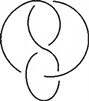2.5. 尖括号多项式

1) 介绍A通道、B通道：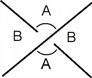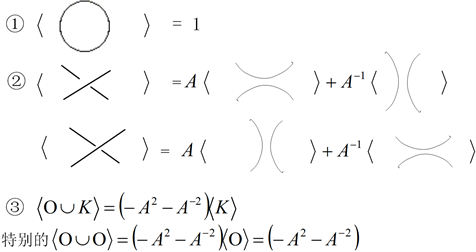2.6. 拧数

2.7. Jones多项式

${S}^{3}$ 中的定向环链。因此，有Jones多项式 $V\left(L\right)$ 与之对应，且满足下列条件：

1) $V\left(L\right)$ 是合痕不变量；

2)有拆接公式： $tV\left({L}_{+}\right)+{t}^{-1}V\left({L}_{-}\right)=\left({t}^{\frac{1}{2}}-{t}^{-\frac{1}{2}}\right)V\left({L}_{0}\right)$

3) $V\left(Ο\right)=1$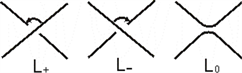2.8. 引理1

$T\left(s\right)$ 是一个排叉结如下图，当s为奇数时， $T\left(s\right)$ 是一个纽结，当s为偶数时， $T\left(s\right)$ 是一个两分支的链环，并且有 $\epsilon \left(s\right)=\frac{s}{|s|}$ 则  ：

$〈T\left(s\right)〉=\left[\underset{i=1}{\overset{|s|-1}{\sum }}{A}^{-\epsilon \left(s\right)\left(2-i\right)}{\left(-{A}^{3}\right)}^{\epsilon \left(s\right)i-s}\right]-{A}^{s+2\epsilon \left(s\right)}$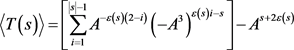2.9. 引理2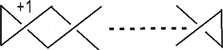$Z>0$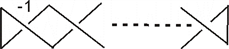$Z<0$

2.10. 引理3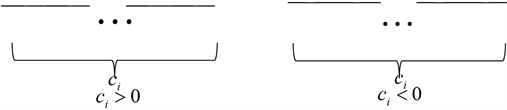${c}_{i}>0$ 时表示正的半扭转，当 ${c}_{i}<0$ 时表示负的半扭转。 $B\left({c}_{1},{c}_{2},\cdots ,{c}_{n}\right)$ 如图所示。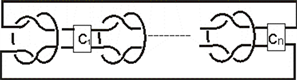${c}_{i}=0\left(i=1,2,\cdots ,n\right)$ 时，此时 $B\left({c}_{1},{c}_{2},\cdots ,{c}_{n}\right)$ 变成 $B\left(0,0,\cdots ,0\right)$。这时所有的半扭转消失，如图所示  。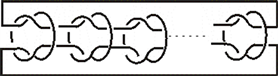$n=1$ 时， $B\left({c}_{1},{c}_{2},\cdots ,{c}_{n}\right)$ 变成 $B\left({c}_{1}\right)$ ，是构成 $B\left({c}_{1},{c}_{2},\cdots ,{c}_{n}\right)$ 的单位元素，也可以理解为由 $B\left(0\right)$ 加入 $|{c}_{1}|$ 个半扭转得到的，如图所示。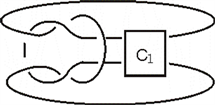3. 定理证明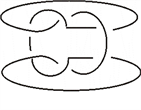$\begin{array}{c}〈B\left(0\right)〉=\left[\underset{i=1}{\overset{2}{\sum }}{A}^{i-2}{\left(-{A}^{3}\right)}^{i-3}-{A}^{5}\right]\left[\underset{i=1}{\overset{2}{\sum }}{A}^{2-i}{\left(-{A}^{3}\right)}^{3-i}-{A}^{-5}\right]\\ =\left({A}^{-7}-{A}^{-3}-{A}^{5}\right)\left({A}^{7}-{A}^{3}-{A}^{-5}\right)\end{array}$

$B\left(0\right)$ 上标记两段弧记为 $\alpha ,\beta$ ，如图所示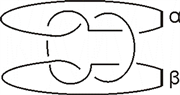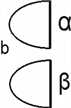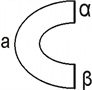$〈B\left(0\right)〉=\left(1-{A}^{4}\right)〈a〉+{A}^{-2}〈b〉$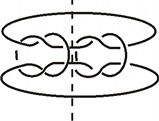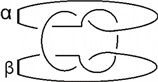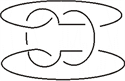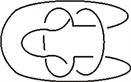$\begin{array}{c}〈B\left(0,0,0\right)〉=\left(1-{A}^{4}\right)〈ΟΟΟ〉+{A}^{-2}〈B\left(0,0\right)〉\\ =\left(1-{A}^{4}\right){\left(-{A}^{2}-{A}^{-2}\right)}^{2}+{A}^{-2}〈B\left(0,0\right)〉\\ =\left(1-{A}^{4}\right){\left(-{A}^{2}-{A}^{-2}\right)}^{2}+{A}^{-2}\left(1-{A}^{4}\right)\left(-{A}^{2}-{A}^{-2}\right)+{\left({A}^{-2}\right)}^{2}〈B\left(0\right)〉\end{array}$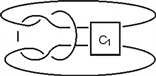①当 $n=1$ 时如上图。

${c}_{1}$ 最右端的交叉点开始打开可得 $〈B\left(c{}_{1}\right)〉={A}^{\epsilon \left({c}_{1}\right)}〈\Lambda 〉+{A}^{-\epsilon \left({c}_{1}\right)}〈B\left({c}_{1}-\epsilon \left({c}_{1}\right)\right)〉$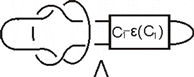$\Lambda$ 可看成如下两部分的连通和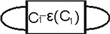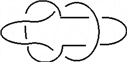$〈B\left({c}_{1}\right)〉={A}^{\epsilon \left({c}_{1}\right)}〈\circ \circ 〉$ $〈$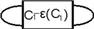$〉$

$〈B\left({c}_{1}\right)〉={A}^{\epsilon \left({c}_{1}\right)}\left(-{A}^{2}-{A}^{-2}\right){\left(-{A}^{3}\right)}^{{c}_{1}-\epsilon \left({c}_{1}\right)}+{A}^{-\epsilon \left({c}_{1}\right)}〈B\left({c}_{1}-\epsilon \left({c}_{1}\right)\right)〉$ (1)

$〈B\left({c}_{1}-\epsilon \left({c}_{1}\right)\right)〉={A}^{\epsilon \left({c}_{1}-\epsilon \left({c}_{1}\right)\right)}\left(-{A}^{2}-{A}^{-2}\right){\left(-{A}^{3}\right)}^{\left({c}_{1}-\epsilon \left({c}_{1}\right)\right)-\epsilon \left({c}_{1}\right)}+{A}^{-\epsilon \left({c}_{1}-\epsilon \left({c}_{1}\right)\right)}〈B\left({c}_{1}-\epsilon \left({c}_{1}\right)-\epsilon \left({c}_{1}\right)\right)〉$

$〈B\left({c}_{1}-\epsilon \left({c}_{1}\right)\right)〉={A}^{\epsilon \left({c}_{1}\right)}\left(-{A}^{2}-{A}^{-2}\right){\left(-{A}^{3}\right)}^{{c}_{1}-2\epsilon \left({c}_{1}\right)}+{A}^{-\epsilon \left({c}_{1}\right)}〈B\left({c}_{1}-2\epsilon \left({c}_{1}\right)\right)〉$

$〈B\left({c}_{1}\right)〉={A}^{\epsilon \left({c}_{1}\right)}\left(-{A}^{2}-{A}^{-2}\right){\left(-{A}^{3}\right)}^{{c}_{1}-\epsilon \left({c}_{1}\right)}+{A}^{0}\left(-{A}^{2}-{A}^{-2}\right){\left(-{A}^{3}\right)}^{{c}_{1}-2\epsilon \left({c}_{1}\right)}+{A}^{-2\epsilon \left({c}_{1}\right)}〈B\left({c}_{1}-2\epsilon \left({c}_{1}\right)\right)〉$

$〈B\left({c}_{1}\right)〉=\left(-{A}^{2}-{A}^{-2}\right)\underset{i=1}{\overset{|{c}_{1}|}{\sum }}{A}^{\epsilon \left({c}_{1}\right)\left(2-i\right)}{\left(-{A}^{3}\right)}^{{c}_{1}-i\epsilon \left({c}_{1}\right)}+{A}^{-|{c}_{1}|\epsilon \left({c}_{1}\right)}〈B\left({c}_{1}-|{c}_{1}|\epsilon \left({c}_{1}\right)\right)〉$

②当 $n=2$ 时如图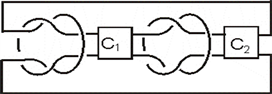${c}_{2}$ 右端的交叉点打开可得： $〈B\left({c}_{1},{c}_{2}\right)〉={A}^{\epsilon \left({c}_{2}\right)}〈L〉+{A}^{-\epsilon \left({c}_{2}\right)}〈B\left({c}_{1},{c}_{2}-\epsilon \left({c}_{2}\right)\right)〉$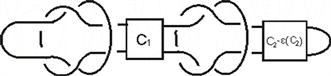L可以看成如下四部分的连通和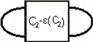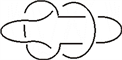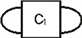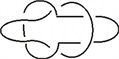$\begin{array}{c}〈B\left({c}_{1},{c}_{2}\right)〉={A}^{\epsilon \left({c}_{2}\right)}\left(-{A}^{2}-{A}^{-2}\right){\left(-{A}^{3}\right)}^{{c}_{1}}\left(-{A}^{2}-{A}^{-2}\right){\left(-{A}^{3}\right)}^{{c}_{2}-\epsilon \left({c}_{2}\right)}+{A}^{-\epsilon \left({c}_{2}\right)}〈B\left({c}_{1},{c}_{2}-\epsilon \left({c}_{2}\right)\right)〉\\ ={A}^{\epsilon \left({c}_{2}\right)}{\left(-{A}^{2}-{A}^{-2}\right)}^{2}{\left(-{A}^{3}\right)}^{{c}_{1}}{\left(-{A}^{3}\right)}^{{c}_{2}-\epsilon \left({c}_{2}\right)}+{A}^{-\epsilon \left({c}_{2}\right)}〈B\left({c}_{1},{c}_{2}-\epsilon \left({c}_{2}\right)\right)〉\end{array}$

$〈B\left({c}_{1},{c}_{2}\right)〉={\left(-{A}^{2}-{A}^{-2}\right)}^{2}{\left(-{A}^{3}\right)}^{{c}_{1}}\left[\underset{i=1}{\overset{|{c}_{2}|}{\sum }}{A}^{\epsilon \left({c}_{2}\right)\left(2-i\right)}{\left(-{A}^{3}\right)}^{{c}_{2}-i\epsilon \left({c}_{2}\right)}\right]+{A}^{-{c}_{2}}〈B\left({c}_{1},0\right)〉$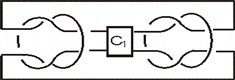${c}_{1}$ 最右面的交叉点开始有

$〈B\left({c}_{1},0\right)〉={A}^{\epsilon \left({c}_{1}\right)}〈H〉+{A}^{-\epsilon \left({c}_{1}\right)}〈B\left({c}_{1}-\epsilon \left({c}_{1}\right),0\right)〉$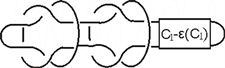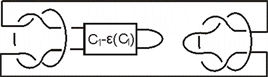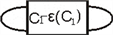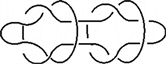$〈H〉=〈\circ \circ \circ 〉〈$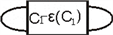$〉$

$\begin{array}{c}〈B\left({c}_{1},0\right)〉={A}^{\epsilon \left({c}_{1}\right)}{\left(-{A}^{2}-{A}^{-2}\right)}^{2}{\left(-{A}^{3}\right)}^{{c}_{1}-\epsilon \left({c}_{1}\right)}+{A}^{-\epsilon \left({c}_{1}\right)}〈B\left({c}_{1}-\epsilon \left({c}_{1}\right),0\right)〉\\ =\left(-{A}^{2}-{A}^{-2}\right)\left[\underset{i=1}{\overset{|{c}_{1}|}{\sum }}{A}^{\epsilon \left({c}_{1}\right)\left(2-i\right)}{\left(-{A}^{3}\right)}^{{c}_{1}-i\epsilon \left({c}_{1}\right)}\right]+{A}^{-{c}_{1}}〈B\left(0,0\right)〉\end{array}$

$\begin{array}{c}〈B\left({c}_{1},{c}_{2}\right)〉={\left(-{A}^{2}-{A}^{-2}\right)}^{2}{\left(-{A}^{3}\right)}^{{c}_{1}}\left[\underset{i=1}{\overset{|{c}_{2}|}{\sum }}{A}^{\epsilon \left({c}_{2}\right)\left(2-i\right)}{\left(-{A}^{3}\right)}^{{c}_{2}-i\epsilon \left({c}_{2}\right)}\right]\\ \text{​}\text{​}\text{​}\text{​}\text{​}\text{​}\text{​}\text{​}\text{​}\text{​}\text{​}\text{​}\text{​}\text{​}\text{​}\text{\hspace{0.17em}}\text{\hspace{0.17em}}+{\left(-{A}^{2}-{A}^{-2}\right)}^{2}{A}^{{c}_{2}}\left[\underset{i=1}{\overset{|{c}_{1}|}{\sum }}{A}^{\epsilon \left({c}_{1}\right)\left(2-i\right)}{\left(-{A}^{3}\right)}^{{c}_{1}-i\epsilon \left({c}_{1}\right)}\right]+{A}^{-{c}_{1}-{c}_{2}}〈B\left(0,0\right)〉\end{array}$

$n=k$ 时，从 ${c}_{k}$ 最右端的交叉点开始打开可得：

$〈B\left({c}_{1},{c}_{2},\cdots ,{c}_{k}\right)〉={A}^{\epsilon \left({c}_{k}\right)}〈D〉+{A}^{-\epsilon \left({c}_{k}\right)}〈B\left({c}_{1},{c}_{2},\cdots ,{c}_{k}-\epsilon \left({c}_{k}\right)\right)〉$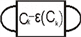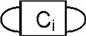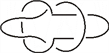$〈D〉={\left(-{A}^{2}-{A}^{-2}\right)}^{k}{\left(-{A}^{3}\right)}^{{c}_{1}+{c}_{2}+\cdots +{c}_{k-1}}{\left(-{A}^{3}\right)}^{{c}_{k}-\epsilon \left(ck\right)}$

$\begin{array}{c}〈B\left({c}_{1},{c}_{2},\cdots ,{c}_{k}\right)〉=\left[{A}^{\epsilon \left({c}_{k}\right)}{\left(-{A}^{2}-{A}^{-2}\right)}^{k}{\left(-{A}^{3}\right)}^{{c}_{1}+{c}_{2}+\cdots +{c}_{k-1}}\right]{\left(-{A}^{3}\right)}^{{c}_{k}-\epsilon \left({c}_{k}\right)}\\ \text{\hspace{0.17em}}\text{\hspace{0.17em}}+{A}^{-\epsilon \left({c}_{k}\right)}〈B\left({c}_{1},{c}_{2},\cdots ,{c}_{k}-\epsilon \left({c}_{k}\right)\right)〉\end{array}$

$\begin{array}{l}〈B\left({c}_{1},{c}_{2},\cdots ,{c}_{k}\right)〉\\ =\left[{\left(-{A}^{2}-{A}^{-2}\right)}^{k}{\left(-{A}^{3}\right)}^{{c}_{1}+{c}_{2}+\cdots +{c}_{k-1}}\right]\underset{i=1}{\overset{|{c}_{k}|}{\sum }}{A}^{\epsilon \left({c}_{k}\right)\left(2-i\right)}{\left(-{A}^{3}\right)}^{{c}_{k}-i\epsilon \left({c}_{k}\right)}+{A}^{-{c}_{k}}〈B\left({c}_{1},{c}_{2},\cdots ,0\right)〉\end{array}$

$B\left({c}_{1},{c}_{2},\cdots ,{c}_{k-1},0\right)$ 中的 ${c}_{k-1}$ 进行一样的操作，从右面的交叉点打开得：

$〈B\left({c}_{1},{c}_{2},\cdots ,{c}_{k-1},0\right)〉={A}^{\epsilon \left({c}_{k-1}\right)}〈W〉+{A}^{-\epsilon \left({c}_{k-1}\right)}〈B\left({c}_{1},{c}_{2}\cdots ,{c}_{k-1}-\epsilon \left({c}_{k-1}\right),0\right)〉$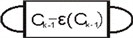$\begin{array}{l}〈B\left({c}_{1},{c}_{2},\cdots ,{c}_{k-1},0\right)〉\\ =\left[{\left(-{A}^{2}-{A}^{-2}\right)}^{k}{\left(-{A}^{3}\right)}^{{c}_{1}+{c}_{2}+\cdots +{c}_{k-2}}\right]{A}^{\epsilon \left({c}_{k-1}\right)}{\left(-{A}^{3}\right)}^{{c}_{k-1}-\epsilon \left({c}_{k-1}\right)}\\ \text{\hspace{0.17em}}\text{\hspace{0.17em}}\text{ }\text{ }+{A}^{-\epsilon \left({c}_{k-1}\right)}〈B\left({c}_{1},{c}_{2},\cdots ,{c}_{k-1}-\epsilon \left({c}_{k-1}\right),0\right)〉\end{array}$

$\begin{array}{l}〈B\left({c}_{1},{c}_{2},\cdots ,{c}_{k-1},0\right)〉\\ =\left[{\left(-{A}^{2}-{A}^{-2}\right)}^{k}{\left(-{A}^{3}\right)}^{{c}_{1}+{c}_{2}+\cdots +{c}_{k-2}}\right]\underset{i=1}{\overset{|{c}_{k-1}|}{\sum }}{A}^{\epsilon \left({c}_{k-1}\right)\left(2-i\right)}{\left(-{A}^{3}\right)}^{{c}_{k-1}-i\epsilon \left({c}_{k-1}\right)}\\ \text{\hspace{0.17em}}\text{\hspace{0.17em}}\text{ }\text{ }+{A}^{-{c}_{k-1}}〈B\left({c}_{1},{c}_{2},\cdots ,0,0\right)〉\end{array}$

$\begin{array}{l}〈B\left({c}_{1},{c}_{2},\cdots ,{c}_{k-2},0\right)〉=\left[{\left(-{A}^{2}-{A}^{-2}\right)}^{k}{\left(-{A}^{3}\right)}^{{c}_{1}+{c}_{2}+\cdots +{c}_{k-3}}\right]\underset{i=1}{\overset{|{c}_{k-2}|}{\sum }}{A}^{\epsilon \left({c}_{k-2}\right)\left(2-i\right)}{\left(-{A}^{3}\right)}^{{c}_{k-2}-i\epsilon \left({c}_{k-2}\right)}\\ \text{\hspace{0.17em}}\text{\hspace{0.17em}}\text{ }\text{ }\text{\hspace{0.17em}}\text{\hspace{0.17em}}\text{\hspace{0.17em}}\text{\hspace{0.17em}}\text{\hspace{0.17em}}\text{\hspace{0.17em}}\text{\hspace{0.17em}}\text{\hspace{0.17em}}\text{\hspace{0.17em}}\text{\hspace{0.17em}}\text{\hspace{0.17em}}\text{\hspace{0.17em}}\text{\hspace{0.17em}}\text{\hspace{0.17em}}\text{\hspace{0.17em}}\text{\hspace{0.17em}}\text{\hspace{0.17em}}\text{\hspace{0.17em}}\text{\hspace{0.17em}}\text{\hspace{0.17em}}\text{\hspace{0.17em}}\text{\hspace{0.17em}}\text{\hspace{0.17em}}\text{\hspace{0.17em}}\text{\hspace{0.17em}}\text{\hspace{0.17em}}\text{\hspace{0.17em}}\text{\hspace{0.17em}}+{A}^{-{c}_{k-2}}〈B\left({c}_{1},{c}_{2},\cdots ,0,0\right)〉\end{array}$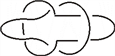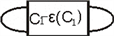$\begin{array}{l}〈B\left({c}_{1},0,0,\cdots ,0\right)〉\\ ={\left(-{A}^{2}-{A}^{-2}\right)}^{k}{A}^{\epsilon \left({c}_{1}\right)}{\left(-{A}^{3}\right)}^{{c}_{1}-\epsilon \left({c}_{1}\right)}+{A}^{-\epsilon \left({c}_{1}\right)}〈B\left({c}_{1}-\epsilon \left({c}_{1}\right),0,\cdots ,0\right)〉\\ ={\left(-{A}^{2}-{A}^{-2}\right)}^{k}\underset{i=1}{\overset{|{c}_{1}|}{\sum }}{A}^{\epsilon \left({c}_{1}\right)\left(2-i\right)}{\left(-{A}^{3}\right)}^{{c}_{1}-i\epsilon \left({c}_{1}\right)}+{A}^{-{c}_{1}}〈B\left(0,0,\cdots ,0\right)〉\end{array}$

$\begin{array}{l}〈B\left({c}_{1},{c}_{2},\cdots ,{c}_{k}\right)〉\\ ={\left(-{A}^{2}-{A}^{-2}\right)}^{k}\underset{u=1}{\overset{k}{\sum }}\left[{\left(-{A}^{3}\right)}^{{c}_{1}+{c}_{2}+\cdots +{c}_{u-1}}{A}^{-{c}_{u+1}-{c}_{k+2}-\cdots -{c}_{k}}\underset{i=1}{\overset{|{c}_{u}|}{\sum }}{A}^{\epsilon \left({c}_{u}\right)\left(2-i\right)}{\left(-{A}^{3}\right)}^{{c}_{u}-i\epsilon \left({c}_{u}\right)}\right]\\ \text{\hspace{0.17em}}\text{\hspace{0.17em}}\text{ }\text{ }+{A}^{-{c}_{1}-{c}_{2}-\cdots -{c}_{k}}〈B\left(0,0,\cdots ,0\right)〉\end{array}$

$u=1$ 时，上式中的 ${\left(-{A}^{3}\right)}^{{c}_{1}+{c}_{2}+\cdots +{c}_{u-1}}{A}^{-{c}_{u+1}-{c}_{u+2}-\cdots -{c}_{k}}$ 仅剩下 ${A}^{-{c}_{2}-{c}_{3}-\cdots -{c}_{k}}$

$u=k$ 时，上式中的 ${\left(-{A}^{3}\right)}^{{c}_{1}+{c}_{2}+\cdots +{c}_{u-1}}{A}^{-{c}_{u+1}-{c}_{u+2}-\cdots -{c}_{k}}$ 仅剩下 ${\left(-{A}^{3}\right)}^{{c}_{1}+{c}_{2}+\cdots +{c}_{k-1}}$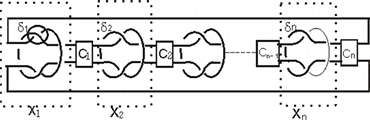i) 计算 ${X}_{i}\left(i=1,2,\cdots ,n\right)$ 部分的拧数

${\delta }_{i-1}=+1$${c}_{i-1}$ 为奇数、 ${\delta }_{i}=+1$ 时，如图所示，有 $\omega \left({X}_{i}\right)=0$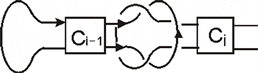${\delta }_{i-1}=+1$${\delta }_{i-1}=-1$${\delta }_{i-1}=-1$${\delta }_{i-1}=+1$${\delta }_{i-1}=+1$${\delta }_{i-1}=-1$${\delta }_{i-1}=-1$、都有 $\omega \left({X}_{i}\right)=0$

ii) 计算所有 ${c}_{i}\left(i=1,2,\cdots ,n\right)$ 部分的拧数

${\delta }_{i}=+1$${c}_{i}>0$ 时，如图所示， ${c}_{i}$ 中每一个交叉点处都是+1，所以有 $\omega \left({c}_{i}\right)={c}_{i}$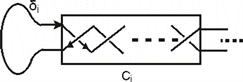$X\left(B\left({\delta }_{1},{c}_{1},{\delta }_{2},{c}_{2},\cdots ,{\delta }_{n},{c}_{n}\right)\right)={\left(-{A}^{3}\right)}^{-\underset{i=1}{\overset{n}{\sum }}{c}_{i}}〈B\left({c}_{1},{c}_{2},\cdots ,{c}_{n}\right)〉$

The Jones Polynomials of Class of Knots[J]. 应用数学进展, 2019, 08(05): 1024-1035. https://doi.org/10.12677/AAM.2019.85117

1. 1. 姜伯驹. 绳圈的数学[M]. 大连: 大连理工大学出版社, 2011: 53-69.

2. 2. 郑淑芳. 几何结构在Brunnian链环的琼斯多项式计算上的应用[D]: [硕士学位论文]. 长春: 东北师范大学, 2009.

3. 3. Adams, C.C. (2004) The Knot Book. W.H. Freeman and Company, New York.

4. 4. 戈鋆. 排叉链环的行列式[J]. 厦门大学学报, 2015, 54(6): 438-479.

5. 5. Jin, X. and Zhang, F. (2004) Zeros of the Jones Polynomials for Families of Pretzel Links. Physica, 333, 183-196. https://doi.org/10.1016/j.physa.2003.10.085

6. 6. Chan, T.H. (2000) Polynomials for a Family Brunnian Links. Journal of Knot Theory and Its Ramifications, 9, 587-609. https://doi.org/10.1142/S0218216500000335

NOTES

*通讯作者